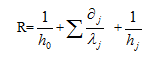﻿ 建筑围护结构热阻及保温计算_求育教学仪器设备厂

## 建筑围护结构热阻及保温计算

（节能）热工计算：
1、围护结构热阻的计算

R=δ/λ

λ—材料导热系数[W/(m.k)]

R=R1+R2+----Rn=δ1/λ1+δ2/λ2+----+δn/λn

δ1、δ2、---δn—各层材料厚度(m)
λ1、λ2、---λn—各层材料导热系数[W/(m.k)]
2、围护结构的传热阻
R0=Ri+R+Re

Re —外表面换热阻(m.k/w)(一般取0.04)
R —围护结构热阻(m.k/w)
3、围护结构传热系数计算
K=1/ R0

Km=(KpFp+Kb1Fb1+Kb2Fb2+ Kb3Fb3 )/( Fp + Fb1+Fb2+Fb3)

Km—外墙的平均传热系数[W/(m.k)]
Kp—外墙主体部位传热系数[W/(m.k)]
Kb1、Kb2、Kb3—外墙周边热桥部位的传热系数[W/(m.k)]
Fp—外墙主体部位的面积
Fb1、Fb2、Fb3—外墙周边热桥部位的面积
4、单一材料热工计算运算式
①厚度δ(m) = 热阻值R(m.k/w) * 导热系数λ[W/(m.k)]
②热阻值R(m.k/w) = 1 / 传热系数K [W/(㎡•K)]
③厚度δ(m) = 导热系数λ[W/(m.k)] / 传热系数K [W/(㎡•K)]
5、围护结构设计厚度的计算

R值和U值是用于衡量建筑材料或装配材料热学性能的两个指标。R值代表建筑材料阻止热量穿过的能力。R值越高，材料的阻热和隔热性能越高。hj 室北墙体内表面空气的对流换热系数,取hj= 23 W·(m2·K) -1
δj为墙体第j层的厚度/m；
λj为墙体第j层的导热系数:W·(m·K)-1
h0为墙体外表面空气的对流换热系数: W·(m2·K) -1,h0=8.7 W·(m2·K) -1

K=1/R
K为墙体的传热系数：W/(m2·K)，试验墙体的K均为0.22 W/(m2·K)，符合国家规定的节能要求。